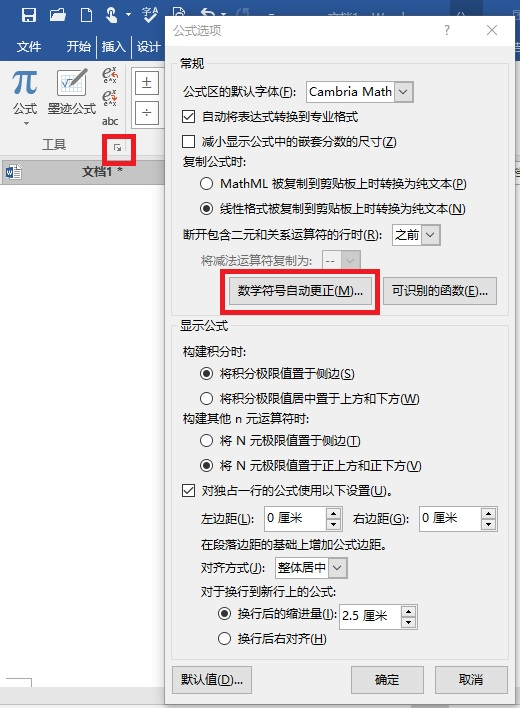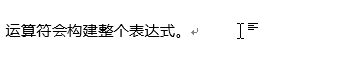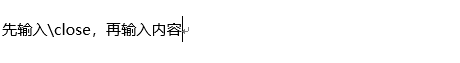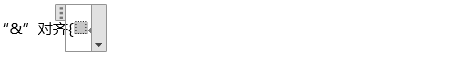# UnicodeMath编码教程

word插入公式不自动斜体的解决办法

# 1. 简介

[La]Tex：$\frac{(a+c)}{d}$

MathML：

<mfrac>
<mrow>
<mi>a</mi>
<mo>+</mo>
<mi>c</mi>
</mrow>
<mi>d</mi>
</mfrac># 2. 编码简单数学表达式

## 2.1 分数

$\frac{abc}{d} 线性格式是abc/d。要强制显示正常大小的线性分数（横着写），可以使用\ /（反斜杠后跟斜杠）。 线性格式(a+c)/d显示为\frac{a+b}{d}。 那问题来了，怎么才能输入\frac{(a+b)}{d}？很简单，再打一对括号：((a+b))/d 另外，分数线样式有三种： 1. “分数斜线”U+2044（可以通过\sdiv输入） 1. “除法斜线”U+2215（\ldiv） 1. 带圆圈的斜线（U+2298,\ndiv） 三种分别显示成 ![](https://img2018.cnblogs.com/blog/1571380/201902/1571380-20190217210719822-101790649.jpg) **提示**：由于分数线/后面不可能跟着运算符，所以/号被定义为取反，即键入/=就可得到≠（类似编程语言中的!=）。类似的有： | Operator | Negated op | Input | |----------|------------|--------------| | < | ≮ | /< | | = | ≠ | /= | | > | ≯ | /> | | ∃ | ∄ | /\exists | | ∈ | ∉ | /\in | | ∋ | ∌ | /\ni | | ∼ | ≁ | /\sim | | ≃ | ≄ | /\simeq | | ≅ | ≇ | /\cong | | ≈ | ≉ | /\approx | | ≍ | ≭ | /\asymp | | ≡ | ≢ | /\equiv | | ≤ | ≰ | /\le | | ≥ | ≱ | /\ge | | ≶ | ≸ | /\lessgtr | | ≷ | ≹ | /\gtrless | | ≽ | ⋡ | /\succeq | | ≺ | ⊀ | /\prec | | ≻ | ⊁ | /\succ | | ≼ | ⋠ | /\preceq | | ⊂ | ⊄ | /\subset | | ⊃ | ⊅ | /\supset | | ⊆ | ⊈ | /\subseteq | | ⊇ | ⊉ | /\supseteq | | ⊑ | ⋢ | /\sqsubseteq | | ⊒ | ⋣ | /\sqsupseteq | 分数的另一个技巧是两个数字之间或斜杠和数字之间的句点或逗号被认为是数字的一部分，而不是终结符。例如，1/3.1416为 \frac{1}{3.1416}，而不是\frac{1}{3}.1416。 ## 2.2 上标和下标 _实现下标：\delta_{\mu\nu} 写为δ_μν。 类似地，^实现上标。所以 a^b 表示 a^b 。 复杂一点，加个括号： \delta_{\mu+\nu} 写为δ_(μ+ν)。 上/下标的嵌套：a_b_c代表a_{b_c}。类似地，a^b^c 代表a^{b^c}。 a^{b_c} 写为a^(b_c)，而不是a^b_c。因为a^b_c(或a_c^b)显示为a^b_c。 稍微复杂的例子，想想这个表达式怎么写？ W^{3\beta}_{\delta_1\rho_2\sigma_3} 其线性格式为W^(3β)_(δ_1ρ_1σ_2)。而在TeX中，需要这样输入 W^{3\beta}_{\delta_1\rho_1\sigma_2} 对于 \alpha_2^3\over\beta_2^3+ \gamma_2^3$

$W_{\delta_1\rho_1\sigma_2}^{3\beta}= U_{\delta_1\rho_1}^{3\beta}+ {1 \over 8\pi^2} \int_{\alpha_1}^{\alpha_2} d\alpha_2’ \left[ {U_{\delta_1\rho_1}^{2\beta}-\alpha_2’ U_{\rho_1\sigma_2}^{1\beta}\over U_{\rho_1\sigma_2}^{0\beta}} \right]$

W_(δ1ρ1σ2)^(3β)=U_(δ1ρ1)^(3β)+1/8π^2 ∫_α1^α2▒dα'_2 [(U_(δ1ρ1)^(2β)-α'_2U_(ρ1σ2)^(1β))/U_(ρ1σ2)^(0β)]

$$W_{\delta_1\rho_1\sigma_2}^{3\beta}= U_{\delta_1\rho_1}^{3\beta}+ {1 \over 8\pi^2} \int_{\alpha_1}^{\alpha_2} d\alpha_2’ \left[ {U_{\delta_1\rho_1}^{2\beta}-\alpha_2’ U_{\rho_1\sigma_2}^{1\beta}\over U_{\rho_1\sigma_2}^{0\beta}} \right]$$


## 2.3 空白（空格）字符使用+这样的其他运算符会构建整个表达式，因为这些运算符明确地终止了操作。# 3. 编码其他数学公式

## 3.1 open/close分隔符

├(\open)和┤(\close)用于标志分隔（类似LaTeX的“\begin” “\end”）。

### 关于大括号方程组（cases）

$$\left|x\right|=\begin{cases}x & x \geq 0\\-x & x < 0\end{cases}$$### 关于缩放

UnicodeMath提供了类似于LaTeX\big \Big \bigg \Bigg的对符号进行放大的用法。

Latex代码：

\Bigg\{
\bigg\{
\Big[
\big(
(x)
\big)
\Big]
\bigg\}
\Bigg\}


$\Bigg\{ \bigg\{ \Big[ \big( (x) \big) \Big] \bigg\} \Bigg\}$

UnicodeMath编码：在├后加一个数字‘0’-‘4’，代表放大值。对照表：

Digit Meaning
0 Don't grow
1 big
2 Big
3 bigg
4 Bigg

├4{├3{├2[├1(├0(x))]}}

### 关于绝对值

UnicodeMath中绝对值直接用竖杆“|”(U+007C)表示（LaTeX是\left| \right|），按空格构建。

## 3.2 转义

█(10&x+&3&y=2@3&x+&13&y=4)

# 4. 输入方法

## 4.6 线性格式自动更正列表

Control word Character Control word Character
\int (U+222B) \underbrace (U+23DF)
\oint (U+222E) \overbrace (U+23DE)
\sum (U+2211) \begin (U+3016)
\prod (U+220F) \end (U+3017)
\funcapply (U+2061) \phantom (U+27E1)
\naryand,\of (U+2592) \box (U+25A1)